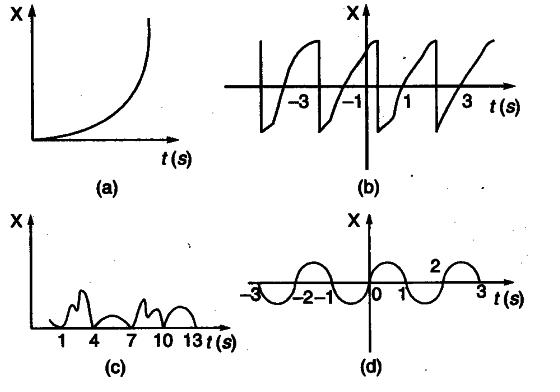# Given below are four x-t plots for linear motion of particle. Which of the plots represent periodic motion ? What is the period of motion (in case of periodic motion)?

Given below are four x-t plots for linear motion of particle. Which of the plots represent periodic motion ? What is the period of motion (in case of periodic motion)?(a) This figure does not represent periodic motion as the motion neither repeats nor comes to mean position.
(b) This figure represents periodic motion with period equal to 2 s.
© This figure does not represents periodic motion because it does not repeat identically.
(d) This figure represents periodic motion having
period equal to 2 s